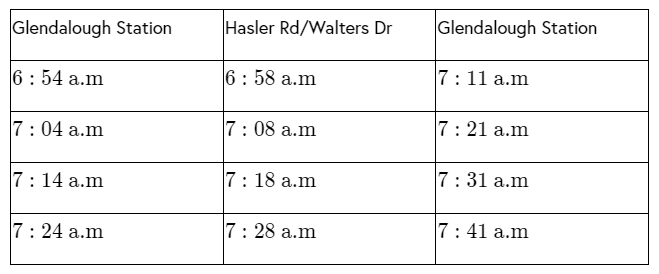# How to read 24 hour time

Last updated: September 21, 2021

## E1.14 A: Calculate times in terms of the $$24$$-hour and $$12$$-hour clocks.

There are two ways of telling the time:

• The $$12$$-hour clock runs from $$1\text{a.m}$$ and $$12$$ noon and then from $$1\text{p.m}$$ to $$12$$ midnight.

• The $$24$$-hour clock uses the numbers $$00:00$$ to $$23:59$$ (midnight $$00:00$$).

### Worked example

Example 1: Write the given  time in the $$24$$-hour format:

1. Lungile wakes up at $$5:40\text{a.m}$$.

2. Simphiwe ate dinner at $$6:59\text{p.m}$$.

Question 1

Step 1: Write the time at which Lungile wakes up.

Lungile wakes up at $$5:40\text{a.m}$$. In the $$24$$-hour format, it is $$5:40$$.

Question 2

Step 2: Write the time at which Simphiwe ate dinner.

Simphiwe ate dinner at $$6:59\text{p.m}$$. In the $$24$$-hour format, it is $$6:59+12=18:59$$

### E1.14 B: Read clocks, dials and timetables

Reading a clock is an easy skill to learn, requiring only a little time and energy. The separate readings of the minute and hour hands help in determining the time.

The watch contains $$2$$ hands: Small hand and large hand. The small hand denotes the number of hours, and the large hand denotes minutes.

For example, if the small hand points to $$1$$. It is $$1$$ o’clock.

A timetable is a schedule for public transportation such as buses, trains and aeroplanes. The timetable tells exactly where the bus, train or aeroplane will be and when it will be there.The above table represents buses' special timetables from Glendalough Station to Hasler Road and Walters Drive and back to Glendalough Station.